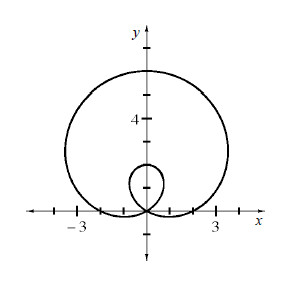### Home > CALC > Chapter 9 > Lesson 9.4.3 > Problem9-139

9-139.

The graph below is called a "limaçon." Use your conclusions from problem 9-130 to find its equation.$r = a + b\operatorname{sin}(θ)$
Notice that the points $(2, 0)$ and $(6,\frac{π}{2})$ are on the graph.
Use these points and substitution to solve for the values of $a$ and $b$.